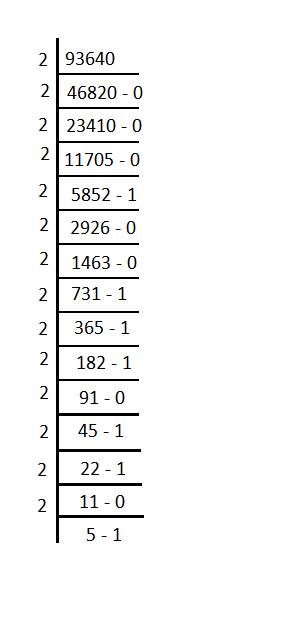# CAT Questions on Base System

0
5607

CAT Questions on Base System:

CAT Holi Offer – Use coupon “HOLI30”

Question 1: $$(245)_{x}+(162)_{x}-(427)_{x}=0$$ in some base x. What is the value of x?

a) 8
b) 9
c) 10
d) Cannot be determined

Question 2: What will be the number of zeroes in$$(2000!)_{34}$$. Here 34 is the base in which the number is written.

a) 122
b) 123
c) 124
d) 125

Question 3: A two digit number A in base 11 is one-third of the number formed by reversing its digits when considered in base 19. How many such numbers are possible?

a) 5
b) 6
c) 7
d) 8

Question 4: How many 3 digit numbers in decimal system are 3 digit numbers in both base 9 and base 11?

a) 625
b) 507
c) 528
d) 608

Question 5: How many 1s exist in the binary equivalent of the decimal number 93640?

a) 6
b) 7
c) 8
d) 9

Solutions for CAT Questions on Base System:

Solutions:

$$(245)_{x}+(162)_{x}-(427)_{x}=0$$
$$=>(2x^2+4x+5)+(x^2+6x+2)-(4x^2+2x+7)=0$$
$$=>x^2-8x=0$$
$$=>x=8$$

34 = 17*2
So we have to find the highest power of 17 in 2000!. We need not find the power of 2 because of 2 will be greater than the power of 17. Thus the power of 17 will act as the limiting value.
Thus the highest power of 17 in 2000! is
[2000/17] + [ 2000/289] + [2000/4913], [] is greatest integer function
= 117 + 6 + 0 = 123
Thus the required number of zeroes is 123.

Let the number be xy.
=> $$(xy)_{11}=\frac{1}{3}(yx)_{19}$$
=> $$y+11x=\frac{1}{3}(x+19y)$$
=> $$3y+33x=x+19y$$
=> $$32x=16y$$ or $$2x=y$$
So, (x,y) can be (2,1)(4,2)(6,3)(8,4)(10,5)
Numbers such as 10, 11, 12.. etc. are represented as alphabets a,b,c etc in higher bases. Thus, the case of (10,5) also satisfies our requirements.
The next pair (12,6) can’t be considered since 12 is not a single digit number in base 11 and thus, all other cases greater than (10,5) can be excluded.

Smallest 3 digit numbers in base 9 is $$1*9^{2} + 0* 9 + 0* 9^{0} = 81$$, Largest 3 digit number in base 9 is $$8*9^{2} + 8*9 + 8*9 = 728$$
Smallest 3 digit number in base 11 is is $$1*11^{2} + 0* 11 + 0* 11^{0} = 121$$
Largest 3 digit number in base 11 is $$10*11^{2} + 10*11 + 10*11^{0}= 1330$$
So 3 digit numbers in base 10 which are also 3 digit numbers in base 9 and base 11 are
from 121 to 728 = 608We know that the binary equivalent of 5 is 101.